# Problem: Part A: Point M is located a distance  from the midpoint between the two wires. Find the magnitude of the magnetic field  created at point M by wire 1.Express your answer in terms of , , and appropriate constants.Part B: Find the magnitude of the net magnetic field  created at point M by both wires.Express your answer in terms of , , and appropriate constants.

###### FREE Expert Solution

Magnetic field due to a current-carrying conductor:

$\overline{){\mathbf{B}}{\mathbf{=}}\frac{{\mathbf{\mu }}_{\mathbf{0}}\mathbf{I}}{\mathbf{2}\mathbf{\pi }\mathbf{r}}}$

Resolving vector components,

(a)

$\begin{array}{rcl}\mathbf{r}& \mathbf{=}& \sqrt{{\mathbf{\left(}\mathbf{2}\mathbf{d}\mathbf{\right)}}^{\mathbf{2}}\mathbf{+}{\mathbf{d}}^{\mathbf{2}}}\\ & \mathbf{=}& \mathbf{d}\sqrt{\mathbf{5}}\end{array}$

${\mathbit{B}}_{\mathbf{1}\mathbf{m}}\mathbf{=}\frac{{\mathbf{\mu }}_{\mathbf{0}}\mathbf{I}}{\mathbf{2}\mathbf{\pi }\mathbf{d}\sqrt{\mathbf{5}}}$###### Problem Details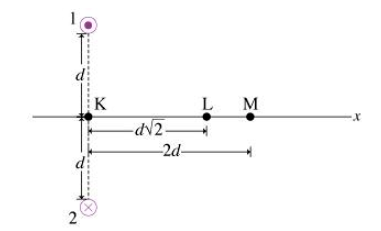Part A: Point M is located a distance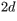from the midpoint between the two wires. Find the magnitude of the magnetic field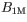created at point M by wire 1.

Express your answer in terms of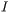,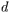, and appropriate constants.

Part B:

Find the magnitude of the net magnetic field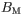created at point M by both wires.

Express your answer in terms of, and appropriate constants.